JMSLTM Numerical Library 7.2.0
com.imsl.stat

## Class ProportionalHazards

• All Implemented Interfaces:
Serializable, Cloneable

```public class ProportionalHazards
extends Object
implements Serializable, Cloneable```
Analyzes survival and reliability data using Cox's proportional hazards model.

Class `ProportionalHazards` computes parameter estimates and other statistics in Proportional Hazards Generalized Linear Models. These models were first proposed by Cox (1972). Two methods for handling ties are allowed. Time-dependent covariates are not allowed. The user is referred to Cox and Oakes (1984), Kalbfleisch and Prentice (1980), Elandt-Johnson and Johnson (1980), Lee (1980), or Lawless (1982), among other texts, for a thorough discussion of the Cox proportional hazards model.

Let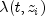represent the hazard rate at time t for observation number i with covariables contained as elements of row vector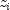. The basic assumption in the proportional hazards model (the proportionality assumption) is that the hazard rate can be written as a product of a time varying function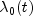, which depends only on time, and a function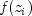, which depends only on the covariable values. The functionused in `ProportionalHazards` is given as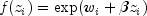where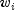is a fixed constant assigned to the observation, and b is a vector of coefficients to be estimated. With this function one obtains a hazard rate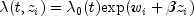. The form ofis not important in proportional hazards models.

The constantsmay be known theoretically. For example, the hazard rate may be proportional to a known length or area, and thecan then be determined from this known length or area. Alternatively, themay be used to fix a subset of the coefficients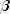(say,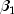) at specified values. Whenis used in this way, constants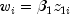are used, while the remaining coefficients inare free to vary in the optimization algorithm. Constants are defined as 0.0 by default. If user-specified constants are desired, use the `setConstantColumn` method to specify which column contains the constant.

With this definition of, the usual partial (or marginal, see Kalbfleisch and Prentice (1980)) likelihood becomes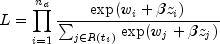where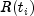denotes the set of indices of observations that have not yet failed at time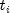(the risk set),denotes the time of failure for the i-th observation,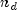is the total number of observations that fail. Right-censored observations (i.e., observations that are known to have survived to time, but for which no time of failure is known) are incorporated into the likelihood through the risk set. Such observations never appear in the numerator of the likelihood. When `setTieOptions` is set to `BRESLOWS_APPROXIMATE` (the default), all observations that are censored at timeare not included in, while all observations that fail at timeare included in.

If it can be assumed that the dependence of the hazard rate upon the covariate values remains the same from stratum to stratum, while the time-dependent term,, may be different in different strata, then `ProportionalHazards` allows the incorporation of strata into the likelihood as follows. Let k index the m strata (set with `setStratumColumn`). Then, the likelihood is given by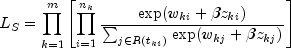In `ProportionalHazards`, the log of the likelihood is maximized with respect to the coefficients. A quasi-Newton algorithm approximating the Hessian via the matrix of sums of squares and cross products of the first partial derivatives is used in the initial iterations. When the change in the log-likelihood from one iteration to the next is less than 100 times the convergence tolerance, Newton-Raphson iteration is used. If, during any iteration, the initial step does not lead to an increase in the log-likelihood, then step halving is employed to find a step that will increase the log-likelihood.

Once the maximum likelihood estimates have been computed, the algorithm computes estimates of a probability associated with each failure. Within stratum k, an estimate of the probability that the i-th observation fails at timegiven the risk set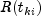is given by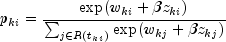A diagnostic "influence" or "leverage" statistic is computed for each noncensored observation as: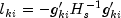where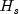is the matrix of second partial derivatives of the log-likelihood, and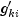is computed as: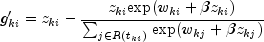Influence statistics are not computed for censored observations.

A "residual" is computed for each of the input observations according to methods given in Cox and Oakes (1984, page 108). Residuals are computed as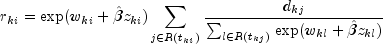where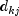is the number of tied failures in group k at time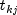. Assuming that the proportional hazards assumption holds, the residuals should approximate a random sample (with censoring) from the unit exponential distribution. By subtracting the expected values, centered residuals can be obtained. (The j-th expected order statistic from the unit exponential with censoring is given as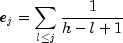where h is the sample size, and censored observations are not included in the summation.)

An estimate of the cumulative baseline hazard within group k is given as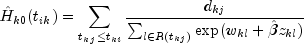The observation proportionality constant is computed as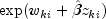Note that the user can use the JDK JAVA Logging API to generate intermediate output for the solver. Accumulated levels of detail correspond to JAVA's FINE, FINER, and FINEST logging levels with FINE yielding the smallest amount of information and FINEST yielding the most. The levels of output yield the following:

 Level Output FINE Logging is enabled, but observational statistics are not printed. FINER All output statistics are printed. FINEST Tracks progress through internal methods.
Example , Serialized Form
• ### Nested Class Summary

Nested Classes
Modifier and Type Class and Description
`static class ` `ProportionalHazards.ClassificationVariableLimitException`
The Classification Variable limit set by the user through ``` setUpperBound``` has been exceeded.
• ### Field Summary

Fields
Modifier and Type Field and Description
`static int` `BRESLOWS_APPROXIMATE`
Breslows approximate method of handling ties.
`static int` `SORTED_AS_PER_OBSERVATIONS`
Failures are assumed to occur in the same order as the observations input in `x`.
• ### Constructor Summary

Constructors
Constructor and Description
```ProportionalHazards(double[][] x, int[] nVarEffects, int[] indEffects)```
Constructor for `ProportionalHazards`.
• ### Method Summary

Methods
Modifier and Type Method and Description
`double[][]` `getCaseStatistics()`
Returns the case statistics for each observation.
`int` `getCensorColumn()`
Returns the column index of `x` containing the optional censoring code for each observation.
`int[]` `getClassValueCounts()`
Returns the number of values taken by each classification variable.
`double[]` `getClassValues()`
Returns the class values taken by each classification variable.
`int` `getConstantColumn()`
Returns the column index of `x` containing the constant to be added to the linear response.
`double` `getConvergenceTol()`
Returns the convergence tolerance used.
`int` `getFrequencyColumn()`
Returns the column index of `x` containing the frequency of response for each observation.
`double[]` `getGradient()`
Returns the inverse of the Hessian times the gradient vector, computed at the initial estimates.
`double[][]` `getHessian()`
Returns the inverse of the Hessian of the negative of the log-likelihood, computed at the initial estimates.
`boolean` `getHessianOption()`
Returns the `boolean` used to indicate whether or not to compute the Hessian and gradient at the initial estimates.
`double[]` `getInitialEstimates()`
Gets the initial parameter estimates.
`double[]` `getLastUpdates()`
`Logger` `getLogger()`
Returns the logger object and enables logging.
`int` `getMaxClass()`
Returns the upper bound used on the sum of the number of distinct values found among the classification variables in `x`.
`double` `getMaximumLikelihood()`
Returns the maximized log-likelihood.
`int` `getMaxIterations()`
Return the maximum number of iterations allowed.
`double[]` `getMeans()`
Returns the means of the design variables.
`int` `getNumberOfCoefficients()`
Returns the number of estimated coefficients in the model.
`int` `getNumberRowsMissing()`
Returns the number of rows of data in `x` that contain missing values in one or more specific columns of `x`.
`double[][]` `getParameterStatistics()`
Returns the parameter estimates and associated statistics.
`int` `getResponseColumn()`
Returns the column index of `x` containing the response time for each observation.
`int` `getStratumColumn()`
Returns the column index of `x` containing the stratum number for each observation.
`int[]` `getStratumNumbers()`
Returns the stratum number used for each observation.
`double` `getStratumRatio()`
Returns the ratio at which a stratum is split into two strata.
`int` `getTiesOption()`
Returns the method used for handling ties.
`double[][]` `getVarianceCovarianceMatrix()`
Returns the estimated asymptotic variance-covariance matrix of the parameters.
`void` `setCensorColumn(int censorIndex)`
Sets the column index of `x` containing the optional censoring code for each observation.
`void` `setClassVarColumns(int[] classVarIndices)`
Sets the column indices of `x` that are the classification variables.
`void` `setConstantColumn(int fixedIndex)`
Sets the column index of `x` containing the constantto be added to the linear response.
`void` `setConvergenceTol(double convergenceTol)`
Set the convergence tolerance.
`void` `setFrequencyColumn(int frequencyIndex)`
Sets the column index of `x` containing the frequency of response for each observation.
`void` `setHessianOption(boolean wantHessian)`
Set the option to have the Hessian and gradient be computed at the initial estimates.
`void` `setInitialEstimates(double[] initialCoef)`
Sets the initial parameter estimates.
`void` `setMaxClass(int maxClass)`
Sets an upper bound on the sum of the number of distinct values found among the classification variables in `x`.
`void` `setMaxIterations(int maxIterations)`
Set the maximum number of iterations allowed.
`void` `setResponseColumn(int responseIndex)`
Sets the column index of `x` containing the response variable.
`void` `setStratumColumn(int stratumIndex)`
Sets the column index of `x` containing the stratification variable.
`void` `setStratumRatio(double stratumRatio)`
Set the ratio at which a stratum is split into two strata.
`void` `setTiesOption(int iTie)`
Sets the method for handling ties.
• ### Methods inherited from class java.lang.Object

`clone, equals, finalize, getClass, hashCode, notify, notifyAll, toString, wait, wait, wait`
• ### Field Detail

• #### BRESLOWS_APPROXIMATE

`public static final int BRESLOWS_APPROXIMATE`
Breslows approximate method of handling ties. See `setTiesOption`.
Constant Field Values
• #### SORTED_AS_PER_OBSERVATIONS

`public static final int SORTED_AS_PER_OBSERVATIONS`
Failures are assumed to occur in the same order as the observations input in `x`. The observations in `x` must be sorted from largest to smallest failure time within each stratum, and grouped by stratum. All observations are treated as if their failure/censoring times were distinct when computing the log-likelihood. See `setTiesOption`.
Constant Field Values
• ### Constructor Detail

• #### ProportionalHazards

```public ProportionalHazards(double[][] x,
int[] nVarEffects,
int[] indEffects)```
Constructor for `ProportionalHazards`.
Parameters:
`x` - a `double` matrix containing the data, including optional data.
`nVarEffects` - an `int` array containing the number of variables associated with each effect in the model.
`indEffects` - an `int` array containing the column numbers of `x` associated with each effect. The first `nVarEffects` elements of `indEffects` contain the column numbers of `x` for the variables in the first effect. The next `nVarEffects` elements of `indEffects` contain the column numbers of `x` for the variables in the second effect, etc.
• ### Method Detail

• #### getCaseStatistics

```public double[][] getCaseStatistics()
throws ProportionalHazards.ClassificationVariableLimitException```
Returns the case statistics for each observation.

There is one row for each observation, and the columns of the returned matrix contain the following:

 Column Statistic `0` Estimated survival probability at the observation time. `1` Estimated observation influence or leverage. `2` A residual estimate. `3` Estimated cumulative baseline hazard rate. `4` Observation proportionality constant.

Returns:
a `double` matrix containing the case statistics.
Throws:
`ProportionalHazards.ClassificationVariableLimitException` - is thrown if the classification variable limit set by the user through ``` setUpperBound``` has been exceeded.
• #### getCensorColumn

`public int getCensorColumn()`
Returns the column index of `x` containing the optional censoring code for each observation.
Returns:
an `int` specifying the column index of `x` containing the optional censoring code for each observation.
• #### getClassValueCounts

```public int[] getClassValueCounts()
throws ProportionalHazards.ClassificationVariableLimitException```
Returns the number of values taken by each classification variable. The i-th element of the returned array is the number of distinct values taken by the i-th classification variable.
Returns:
an `int` array containing the number of values taken by each classification variable.
Throws:
`ProportionalHazards.ClassificationVariableLimitException` - is thrown if the classification variable limit set by the user through ``` setUpperBound``` has been exceeded.
• #### getClassValues

```public double[] getClassValues()
throws ProportionalHazards.ClassificationVariableLimitException```
Returns the class values taken by each classification variable. For description purposes, let
nclval = `getClassValueCounts()`. Then the first nclval elements contain the values for the first classification variable, the next nclval elements contain the values for the second classification variable, etc.
Returns:
a `double` array containing the values taken by each classification variable.
Throws:
`ProportionalHazards.ClassificationVariableLimitException` - is thrown if the classification variable limit set by the user through ``` setUpperBound``` has been exceeded.
• #### getConstantColumn

`public int getConstantColumn()`
Returns the column index of `x` containing the constant to be added to the linear response.
Returns:
an `int` specifying the column index of `x` containing the constant to be added to the linear response.
• #### getConvergenceTol

`public double getConvergenceTol()`
Returns the convergence tolerance used.
Returns:
a `double` specifying the convergence tolerance used.
• #### getFrequencyColumn

`public int getFrequencyColumn()`
Returns the column index of `x` containing the frequency of response for each observation.
Returns:
an `int` specifying the column index of `x` containing the frequency of response for each observation.

```public double[] getGradient()
throws ProportionalHazards.ClassificationVariableLimitException```
Returns the inverse of the Hessian times the gradient vector, computed at the initial estimates.

Note that the `setHessianOption` method must be invoked with `wantHessian` set to `true` and the `setInitialEstimates` method must be invoked prior to invoking this method. Otherwise, the method throws an `IllegalStateException` exception.

Returns:
a `double` array containing the inverse of the Hessian times the gradient vector, computed at the initial estimates.
Throws:
`ProportionalHazards.ClassificationVariableLimitException` - is thrown if the classification variable limit set by the user through ``` setUpperBound``` has been exceeded.
• #### getHessian

```public double[][] getHessian()
throws ProportionalHazards.ClassificationVariableLimitException```
Returns the inverse of the Hessian of the negative of the log-likelihood, computed at the initial estimates.

Note that the `setHessianOption` method must be invoked with `wantHessian` set to `true` and the `setInitialEstimates` method must be invoked prior to invoking this method.

Otherwise, the method throws an `IllegalStateException` exception.

Returns:
a `double` matrix containing the inverse of the Hessian of the negative of the log-likelihood, computed at the initial estimates.
Throws:
`ProportionalHazards.ClassificationVariableLimitException` - is thrown if the classification variable limit set by the user through ``` setUpperBound``` has been exceeded.
• #### getHessianOption

`public boolean getHessianOption()`
Returns the `boolean` used to indicate whether or not to compute the Hessian and gradient at the initial estimates.
Returns:
a `boolean` specifying whether or not the Hessian and gradient are to be computed at the initial estimates. A return value equal to `true` indicates that the Hessian and gradient are to be computed.
• #### getInitialEstimates

```public double[] getInitialEstimates()
throws ProportionalHazards.ClassificationVariableLimitException```
Gets the initial parameter estimates.
Returns:
a `double` array containing the initial parameter estimates.
Throws:
`ProportionalHazards.ClassificationVariableLimitException` - is thrown if the classification variable limit set by the user through ``` setUpperBound``` has been exceeded.

```public double[] getLastUpdates()
throws ProportionalHazards.ClassificationVariableLimitException```
Returns:
a `double` array containing the last parameter updates (excluding step halvings).
Throws:
`ProportionalHazards.ClassificationVariableLimitException` - is thrown if the classification variable limit set by the user through ``` setUpperBound``` has been exceeded.
• #### getLogger

`public Logger getLogger()`
Returns the logger object and enables logging.
Returns:
a `java.util.logging.Logger` object, if present, or `null`.
• #### getMaxClass

`public int getMaxClass()`
Returns the upper bound used on the sum of the number of distinct values found among the classification variables in `x`.
Returns:
an `int` representing the upper bound used on the sum of the number of distinct values found among the classification variables in `x`.
• #### getMaximumLikelihood

```public double getMaximumLikelihood()
throws ProportionalHazards.ClassificationVariableLimitException```
Returns the maximized log-likelihood.

The log-likelihood is fully described in the `ProportionalHazards` class description.

Returns:
a `double` representing the maximized log-likelihood
Throws:
`ProportionalHazards.ClassificationVariableLimitException` - is thrown if the classification variable limit set by the user through ``` setUpperBound``` has been exceeded.
• #### getMaxIterations

`public int getMaxIterations()`
Return the maximum number of iterations allowed.
Returns:
an `int` specifying the maximum number of iterations allowed.
• #### getMeans

```public double[] getMeans()
throws ProportionalHazards.ClassificationVariableLimitException```
Returns the means of the design variables.
Returns:
a `double` array containing the means of the design variables.
Throws:
`ProportionalHazards.ClassificationVariableLimitException` - is thrown if the classification variable limit set by the user through ``` setUpperBound``` has been exceeded.
• #### getNumberOfCoefficients

```public int getNumberOfCoefficients()
throws ProportionalHazards.ClassificationVariableLimitException```
Returns the number of estimated coefficients in the model.
Returns:
an `int` scalar representing the number of estimated coefficients in the model.
Throws:
`ProportionalHazards.ClassificationVariableLimitException` - is thrown if the classification variable limit set by the user through ``` setUpperBound``` has been exceeded.
• #### getNumberRowsMissing

```public int getNumberRowsMissing()
throws ProportionalHazards.ClassificationVariableLimitException```
Returns the number of rows of data in `x` that contain missing values in one or more specific columns of `x`.
Returns:
an `int` scalar representing the number of rows of data in `x` that contain missing values in one or more specific columns of `x`.
Throws:
`ProportionalHazards.ClassificationVariableLimitException` - is thrown if the classification variable limit set by the user through ``` setUpperBound``` has been exceeded.
• #### getParameterStatistics

```public double[][] getParameterStatistics()
throws ProportionalHazards.ClassificationVariableLimitException```
Returns the parameter estimates and associated statistics.

There is one row for each coefficient, and the columns of the returned matrix contain the following:

 Column Statistic `0` The coefficient estimate,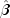`1` Estimated standard deviation of the estimated coefficient `2` Asymptotic normal score for testing that the coefficient is zero against the two-sided alternative `3` p-value associated with the normal score in column 2

Returns:
a `double` matrix containing the parameter estimates and associated statistics.
Throws:
`ProportionalHazards.ClassificationVariableLimitException` - is thrown if the classification variable limit set by the user through ``` setUpperBound``` has been exceeded.
• #### getResponseColumn

`public int getResponseColumn()`
Returns the column index of `x` containing the response time for each observation.
Returns:
an `int` specifying the column index of `x` containing the response time for each observation.
• #### getStratumColumn

`public int getStratumColumn()`
Returns the column index of `x` containing the stratum number for each observation.
Returns:
an `int` specifying the column index of `x` containing the stratum number for each observation.
• #### getStratumNumbers

```public int[] getStratumNumbers()
throws ProportionalHazards.ClassificationVariableLimitException```
Returns the stratum number used for each observation. If `stratumRatio` is not -1.0, additional "strata" (other than those specified by column `groupIndex` of `x` set via the `setStratumColumn` method) may be generated. The array also contains a record of the generated strata. See the `ProportionalHazards` class description for more detail.
Returns:
an `int` array containing the stratum number used for each observation.
Throws:
`ProportionalHazards.ClassificationVariableLimitException` - is thrown if the classification variable limit set by the user through ``` setUpperBound``` has been exceeded.
• #### getStratumRatio

`public double getStratumRatio()`
Returns the ratio at which a stratum is split into two strata.
Returns:
a `double` specifying the ratio at which a stratum is split into two strata.
• #### getTiesOption

`public int getTiesOption()`
Returns the method used for handling ties.
Returns:
an `int` specifying the method to be used in handling ties as indicated by the value in the following table:

 `Value` Method `BRESLOWS_APPROXIMATE` Breslow's approximate method. This is the default. `SORTED_AS_PER_OBSERVATIONS` Failures are assumed to occur in the same order as the observations input in `x`. The observations in `x` must be sorted from largest to smallest failure time within each stratum, and grouped by stratum. All observations are treated as if their failure/censoring times were distinct when computing the log-likelihood.

• #### getVarianceCovarianceMatrix

```public double[][] getVarianceCovarianceMatrix()
throws ProportionalHazards.ClassificationVariableLimitException```
Returns the estimated asymptotic variance-covariance matrix of the parameters.
Returns:
a `double` matrix containing the estimated asymptotic variance-covariance matrix of the parameters.
Throws:
`ProportionalHazards.ClassificationVariableLimitException` - is thrown if the classification variable limit set by the user through ``` setUpperBound``` has been exceeded.
• #### setCensorColumn

`public void setCensorColumn(int censorIndex)`
Sets the column index of `x` containing the optional censoring code for each observation.

If `x[i][censorIndex]` equals 0, the failure time `x[i][responseIndex]` is treated as an exact time of failure. Otherwise, it is treated as right-censored time. By default, it is assumed that there is no censor code column in `x` and all observations are assumed to be exact failure times.

Parameters:
`censorIndex` - an `int` specifying the column index of `x` containing the optional censoring code for each observation.
• #### setClassVarColumns

`public void setClassVarColumns(int[] classVarIndices)`
Sets the column indices of `x` that are the classification variables.
Parameters:
`classVarIndices` - an `int` array containing the column numbers of `x` that are the classification variables. By default it is assumed there are no classification variables.
• #### setConstantColumn

`public void setConstantColumn(int fixedIndex)`
Sets the column index of `x` containing the constantto be added to the linear response.

The linear response is taken to be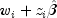whereis the observation constant,is the observation design row vector, andis the vector of estimated parameters. The "fixed" constant allows one to test hypotheses about parameters via the log-likelihoods. If this method is not called, it is assumed that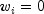for all observations.

Parameters:
`fixedIndex` - an `int` specifying the column index of `x` containing the constant to be added to the linear response.
• #### setConvergenceTol

`public void setConvergenceTol(double convergenceTol)`
Set the convergence tolerance.

Convergence is assumed when the relative change in the maximum likelihood from one iteration to the next is less than `convergenceTol`. If `convergenceTol` is zero, `convergenceTol` = 0.0001 is assumed. The default value is 0.0001.

Parameters:
`convergenceTol` - a `double` specifying the convergence tolerance.
• #### setFrequencyColumn

`public void setFrequencyColumn(int frequencyIndex)`
Sets the column index of `x` containing the frequency of response for each observation.

By default it is assumed that there is no frequency response column recorded in `x`. Each observation in the data array is assumed to be for a single failure; that is, the frequency of response for each observation is 1.

Parameters:
`frequencyIndex` - an `int` specifying the column index of `x` containing the frequency of response for each observation.
• #### setHessianOption

`public void setHessianOption(boolean wantHessian)`
Set the option to have the Hessian and gradient be computed at the initial estimates.
Parameters:
`wantHessian` - a `boolean` specifying whether or not the Hessian and gradient are to be computed at the initial estimates. If this option is set to `true` the user must set the initial estimates via the `setInitialEstimates` method. By default the Hessian and gradient are not computed at the initial estimates.
• #### setInitialEstimates

`public void setInitialEstimates(double[] initialCoef)`
Sets the initial parameter estimates.

Care should be taken to ensure that the supplied estimates for the model coefficientscorrespond to the generated covariate vector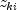.

Parameters:
`initialCoef` - a `double` array containing the initial parameter estimates. By default the initial parameter estimates are all 0.0.
• #### setMaxClass

`public void setMaxClass(int maxClass)`
Sets an upper bound on the sum of the number of distinct values found among the classification variables in `x`.

For example, if the model consisted of of two class variables, one with the values {1, 2, 3, 4} and a second with the values {0, 1}, then the total number of different classification values is 4 + 2 = 6, and `maxClass`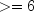. The default value is the number of observations in `x`.

Parameters:
`maxClass` - an `int` representing an upper bound on the sum of the number of distinct values found among the classification variables in `x`.
• #### setMaxIterations

`public void setMaxIterations(int maxIterations)`
Set the maximum number of iterations allowed.
Parameters:
`maxIterations` - an `int` specifying the maximum number of iterations allowed. The default value is 30.
• #### setResponseColumn

`public void setResponseColumn(int responseIndex)`
Sets the column index of `x` containing the response variable.

For point observations, `x[i][responseIndex]` contains the time of the i-th event. For right-censored observations, `x[i][responseIndex]` contains the right-censoring time. Note that because `ProportionalHazards` only uses the order of the events, negative "times" are allowed. By default `responseIndex = 0`.

Parameters:
`responseIndex` - an `int` specifying the column index of `x` containing the response variable.
• #### setStratumColumn

`public void setStratumColumn(int stratumIndex)`
Sets the column index of `x` containing the stratification variable.

Column `stratumIndex` of `x` contains a unique value for each stratum in the data. The risk set for an observation is determined by its stratum. By default it is assumed that all obvservations are from one statum.

Parameters:
`stratumIndex` - an `int` specifying the column index of `x` containing the stratification variable.
• #### setStratumRatio

`public void setStratumRatio(double stratumRatio)`
Set the ratio at which a stratum is split into two strata.

Let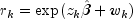be the observation proportionality constant, where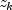is the design row vector for the k-th observation and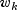is the optional fixed parameter specified by. Let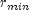be the minimum value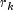in a stratum, where, for failed observations, the minimum is over all times less than or equal to the time of occurrence of the k-th observation. Let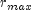be the maximum value offor the remaining observations in the group. Then, if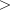`stratumRatio`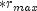, the observations in the group are divided into two groups at k. The default value of `stratumRatio` = 1000 is usually good.

Set `stratumRatio` to any negative value if no division into strata is to be made.

Parameters:
`stratumRatio` - a `double` specifying the ratio at which a stratum is split into two strata.
• #### setTiesOption

`public void setTiesOption(int iTie)`
Sets the method for handling ties.
Parameters:
`iTie` - an `int` specifying the method to be used in handling ties. It can be either `BRESLOWS_APPROXIMATE` or `SORTED_AS_PER_OBSERVATIONS`.

 `iTie` Method `BRESLOWS_APPROXIMATE` Breslow's approximate method. This is the default. `SORTED_AS_PER_OBSERVATIONS` Failures are assumed to occur in the same order as the observations input in `x`. The observations in `x` must be sorted from largest to smallest failure time within each stratum, and grouped by stratum. All observations are treated as if their failure/censoring times were distinct when computing the log-likelihood.

By default, `iTie` is `BRESLOWS_APPROXIMATE`.

JMSLTM Numerical Library 7.2.0